Gurchetan1000 Singh — Published On February 8, 2018 and Last Modified On May 17th, 2023

## Introduction

Most of us would have heard about the new buzz in the market i.e. Cryptocurrency. Many of us would have invested in their coins too. But is investing money in such a volatile currency safe? How can we make sure that investing in these coins now would surely generate a healthy profit in the future? We can’t be sure but we can surely generate an approximate value based on the previous prices. Time series modeling is one way to predict them.

Source: Bitcoin

Besides Cryptocurrencies, there are multiple important areas where time series forecasting is used – forecasting Sales, Call Volume in a Call Center, Solar activity, Ocean tides, Stock market behaviour, and many others.

Assume the Manager of a hotel wants to predict how many visitors should he expect next year to accordingly adjust the hotel’s inventories and make a reasonable guess of the hotel’s revenue. Based on the data of the previous years/months/days, (S)he can use time series forecasting and get an approximate value of the visitors. Forecasted value of visitors will help the hotel to manage the resources and plan things accordingly.In this article, we will learn about multiple forecasting techniques and compare them by implementing on a dataset. We will go through different techniques and see how to use these methods to improve score.

Let’s get started!

## Understanding the Problem Statement and Dataset

We are provided with a Time Series problem involving prediction of number of commuters of JetRail, a new high speed rail service by Unicorn Investors. We are provided with 2 years of data(Aug 2012-Sept 2014) and using this data we have to forecast the number of commuters for next 7 months.

import pandas as pd
import numpy as np
import matplotlib.pyplot as plt
#Importing data

#Printing tail
df.tail()

Python Code:

As seen from the print statements above, we are given 2 years of data(2012-2014) at hourly level with the number of commuters travelling and we need to estimate the number of commuters for future.

In this article, I’m subsetting and aggregating dataset at daily basis to explain the different methods.

• Subsetting the dataset from (August 2012 – Dec 2013)
• Creating train and test file for modeling. The first 14 months (August 2012 – October 2013) are used as training data and next 2 months (Nov 2013 – Dec 2013) as testing data.
• Aggregating the dataset at daily basis
#Subsetting the dataset
#Index 11856 marks the end of year 2013
df = pd.read_csv('train.csv', nrows = 11856)

#Creating train and test set
#Index 10392 marks the end of October 2013
train=df[0:10392]
test=df[10392:]

#Aggregating the dataset at daily level
df.Timestamp = pd.to_datetime(df.Datetime,format='%d-%m-%Y %H:%M')
df.index = df.Timestamp
df = df.resample('D').mean()
train.Timestamp = pd.to_datetime(train.Datetime,format='%d-%m-%Y %H:%M')
train.index = train.Timestamp
train = train.resample('D').mean()
test.Timestamp = pd.to_datetime(test.Datetime,format='%d-%m-%Y %H:%M')
test.index = test.Timestamp
test = test.resample('D').mean()


Let’s visualize the data (train and test together) to know how it varies over a time period.

#Plotting data
train.Count.plot(figsize=(15,8), title= 'Daily Ridership', fontsize=14)
test.Count.plot(figsize=(15,8), title= 'Daily Ridership', fontsize=14)
plt.show()

## Installing library(statsmodels)

The library which I have used to perform Time series forecasting is statsmodels. You need to install it before applying few of the given approaches. statsmodels might already be installed in your python environment but it doesn’t support forecasting methods. We will clone it from their repository and install using the source code. Follow these steps :-

1. Use pip freeze to check if it’s already installed in your environment.
2. If already present, remove it using “conda remove statsmodels”
3. Clone the statsmodels repository using “git clone git://github.com/statsmodels/statsmodels.git”. Initialise the Git using “git init” before cloning.
4. Change the directory to statsmodels using “cd statsmodels”
5. Build the setup file using “python setup.py build”
6. Install it using “python setup.py install”
7. Exit the bash/terminal
8. Restart the bash/terminal in your environment, open python and execute “from statsmodels.tsa.api import ExponentialSmoothing” to verify.

## Method 1: Naive Forecast Python

Consider the graph given below. Let’s assume that the y-axis depicts the price of a coin and x-axis depicts the time (days).

We can infer from the graph that the price of the coin is stable from the start. Many a times we are provided with a dataset, which is stable throughout it’s time period. If we want to forecast the price for the next day, we can simply take the last day value and estimate the same value for the next day. Such forecasting technique which assumes that the next expected point is equal to the last observed point is called Naive Method.

Now we will implement the Naive method to forecast the prices for test data.

dd= np.asarray(train.Count)
y_hat = test.copy()
y_hat['naive'] = dd[len(dd)-1]
plt.figure(figsize=(12,8))
plt.plot(train.index, train['Count'], label='Train')
plt.plot(test.index,test['Count'], label='Test')
plt.plot(y_hat.index,y_hat['naive'], label='Naive Forecast')
plt.legend(loc='best')
plt.title("Naive Forecast")
plt.show()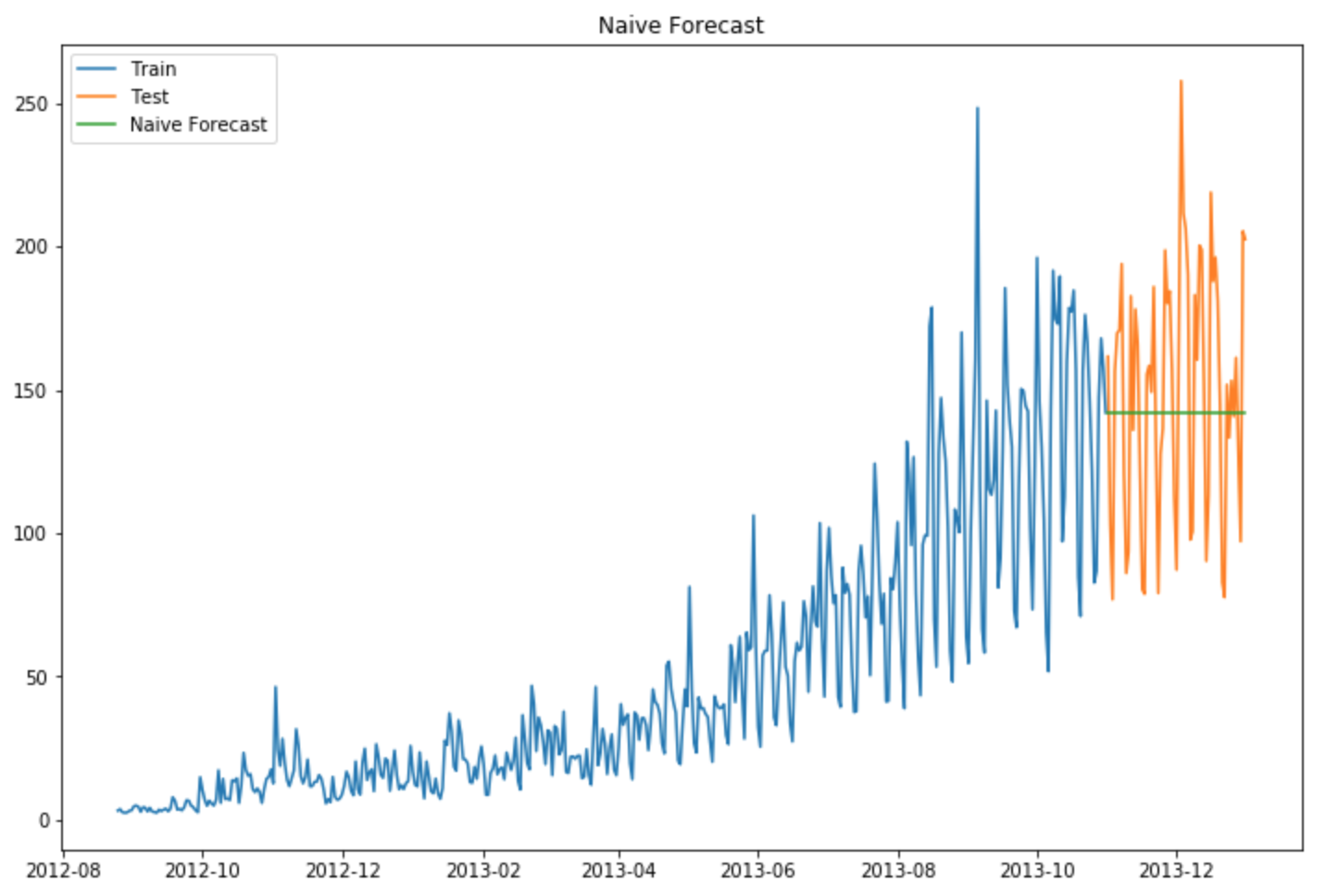We will now calculate RMSE to check to accuracy of our model on test data set.

from sklearn.metrics import mean_squared_error
from math import sqrt
rms = sqrt(mean_squared_error(test.Count, y_hat.naive))
print(rms)

RMSE = 43.9164061439

We can infer from the RMSE value and the graph above, that Naive method isn’t suited for datasets with high variability. It is best suited for stable datasets. We can still improve our score by adopting different techniques. Now we will look at another technique and try to improve our score.

## Method 2: Simple Average

Consider the graph given below. Let’s assume that the y-axis depicts the price of a coin and x-axis depicts the time(days).

We can infer from the graph that the price of the coin is increasing and decreasing randomly by a small margin, such that the average remains constant. Many a times we are provided with a dataset, which though varies by a small margin throughout it’s time period, but the average at each time period remains constant. In such a case we can forecast the price of the next day somewhere similar to the average of all the past days.

Such forecasting technique which forecasts the expected value equal to the average of all previously observed points is called Simple Average technique.

We take all the values previously known, calculate the average and take it as the next value. Of course it won’t be it exact, but somewhat close. As a forecasting method, there are actually situations where this technique works the best.

y_hat_avg = test.copy()
y_hat_avg['avg_forecast'] = train['Count'].mean()
plt.figure(figsize=(12,8))
plt.plot(train['Count'], label='Train')
plt.plot(test['Count'], label='Test')
plt.plot(y_hat_avg['avg_forecast'], label='Average Forecast')
plt.legend(loc='best')
plt.show()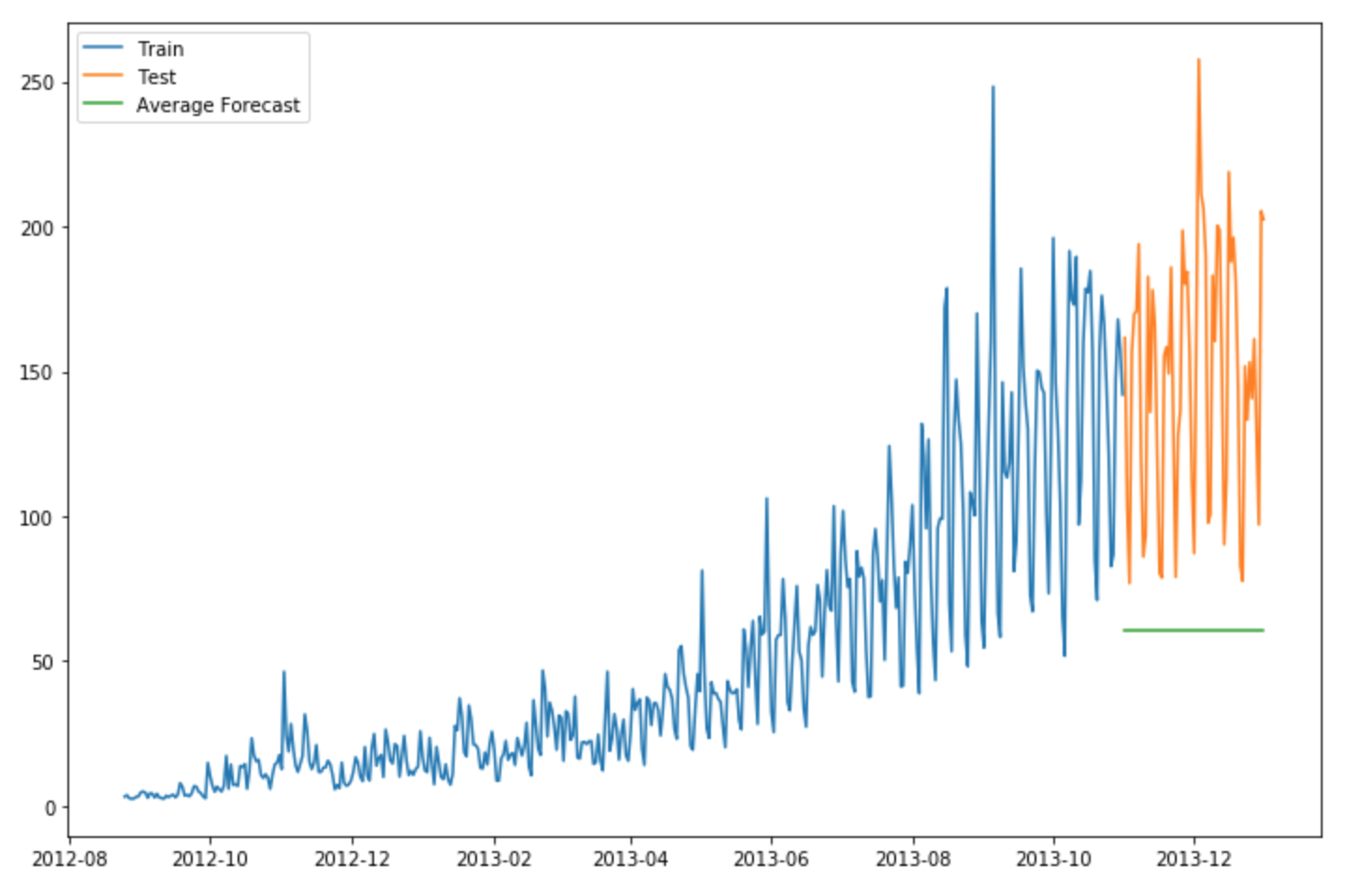We will now calculate RMSE to check to accuracy of our model.

rms = sqrt(mean_squared_error(test.Count, y_hat_avg.avg_forecast))
print(rms)

RMSE = 109.545990803

We can see that this model didn’t improve our score. Hence we can infer from the score that this method works best when the average at each time period remains constant. Though the score of Naive method is better than Average method, but this does not mean that the Naive method is better than Average method on all datasets. We should move step by step to each model and confirm whether it improves our model or not.

## Method 3: Moving Average Time Series Forecasting Python

Consider the graph given below. Let’s assume that the y-axis depicts the price of a coin and x-axis depicts the time(days).

We can infer from the graph that the prices of the coin increased some time periods ago by a big margin but now they are stable. Many a times we are provided with a dataset, in which the prices/sales of the object increased/decreased sharply some time periods ago. In order to use the  previous Average method, we have to use the mean of all the previous data, but using all the previous data doesn’t sound right.

Using the prices of the initial period would highly affect the forecast for the next period. Therefore as an improvement over simple average, we will take the average of the prices for last few time periods only. Obviously the thinking here is that only the recent values matter. Such forecasting technique which uses window of time period for calculating the average is called Moving Average technique. Calculation of the moving average involves what is sometimes called a “sliding window” of size n.

Using a simple moving average model, we forecast the next value(s) in a time series based on the average of a fixed finite number ‘p’ of the previous values. Thus, for all i > p

A moving average can actually be quite effective, especially if you pick the right p for the series.

y_hat_avg = test.copy()
y_hat_avg['moving_avg_forecast'] = train['Count'].rolling(60).mean().iloc[-1]
plt.figure(figsize=(16,8))
plt.plot(train['Count'], label='Train')
plt.plot(test['Count'], label='Test')
plt.plot(y_hat_avg['moving_avg_forecast'], label='Moving Average Forecast')
plt.legend(loc='best')
plt.show()

We chose the data of last 2 months only. We will now calculate RMSE to check to accuracy of our model.

rms = sqrt(mean_squared_error(test.Count, y_hat_avg.moving_avg_forecast))
print(rms)
RMSE = 46.7284072511

We can see that Naive method outperforms both Average method and Moving Average method for this dataset. Now we will look at Simple Exponential Smoothing method and see how it performs.

An advancement over Moving average method is Weighted moving average method. In the Moving average method as seen above, we equally weigh the past ‘n’ observations. But we might encounter situations where each of the observation from the past ‘n’ impacts the forecast in a different way. Such a technique which weighs the past observations differently is called Weighted Moving Average technique.

A weighted moving average is a moving average where within the sliding window values are given different weights, typically so that more recent points matter more. Ins

tead of selecting a window size, it requires a list of weights (which should add up to 1). For example if we pick [0.40, 0.25, 0.20, 0.15] as weights, we would be giving 40%, 25%, 20% and 15% to the last 4 points respectively.

## Method 4: Simple Exponential Smoothing

After we have understood the above methods, we can note that both Simple average and Weighted moving average lie on completely opposite ends. We would need something between these two extremes approaches which takes into account all the data while weighing the data points differently. For example it may be sensible to attach larger weights to more recent observations than to observations from the distant past. The technique which works on this principle is called Simple exponential smoothing. Forecasts are calculated using weighted averages where the weights decrease exponentially as observations come from further in the past, the smallest weights are associated with the oldest observations:

where 0≤ α ≤1 is the smoothing parameter.

The one-step-ahead forecast for time T+1 is a weighted average of all the observations in the series y1,…,yT. The rate at which the weights decrease is controlled by the parameter α.

If you stare at it just long enough, you will see that the expected value ŷx is the sum of two products: α⋅yt and (1−α)⋅ŷ t-1.

Hence, it can also be written as :

So essentially we’ve got a weighted moving average with two weights: α and 1−α.

As we can see, 1−α is multiplied by the previous expected value ŷ x−1 which makes the expression recursive. And this is why this method is called Exponential. The forecast at time t+1 is equal to a weighted average between the most recent observation yt and the most recent forecast ŷ t|t−1.

from statsmodels.tsa.api import ExponentialSmoothing, SimpleExpSmoothing, Holt
y_hat_avg = test.copy()
fit2 = SimpleExpSmoothing(np.asarray(train['Count'])).fit(smoothing_level=0.6,optimized=False)
y_hat_avg['SES'] = fit2.forecast(len(test))
plt.figure(figsize=(16,8))
plt.plot(train['Count'], label='Train')
plt.plot(test['Count'], label='Test')
plt.plot(y_hat_avg['SES'], label='SES')
plt.legend(loc='best')
plt.show()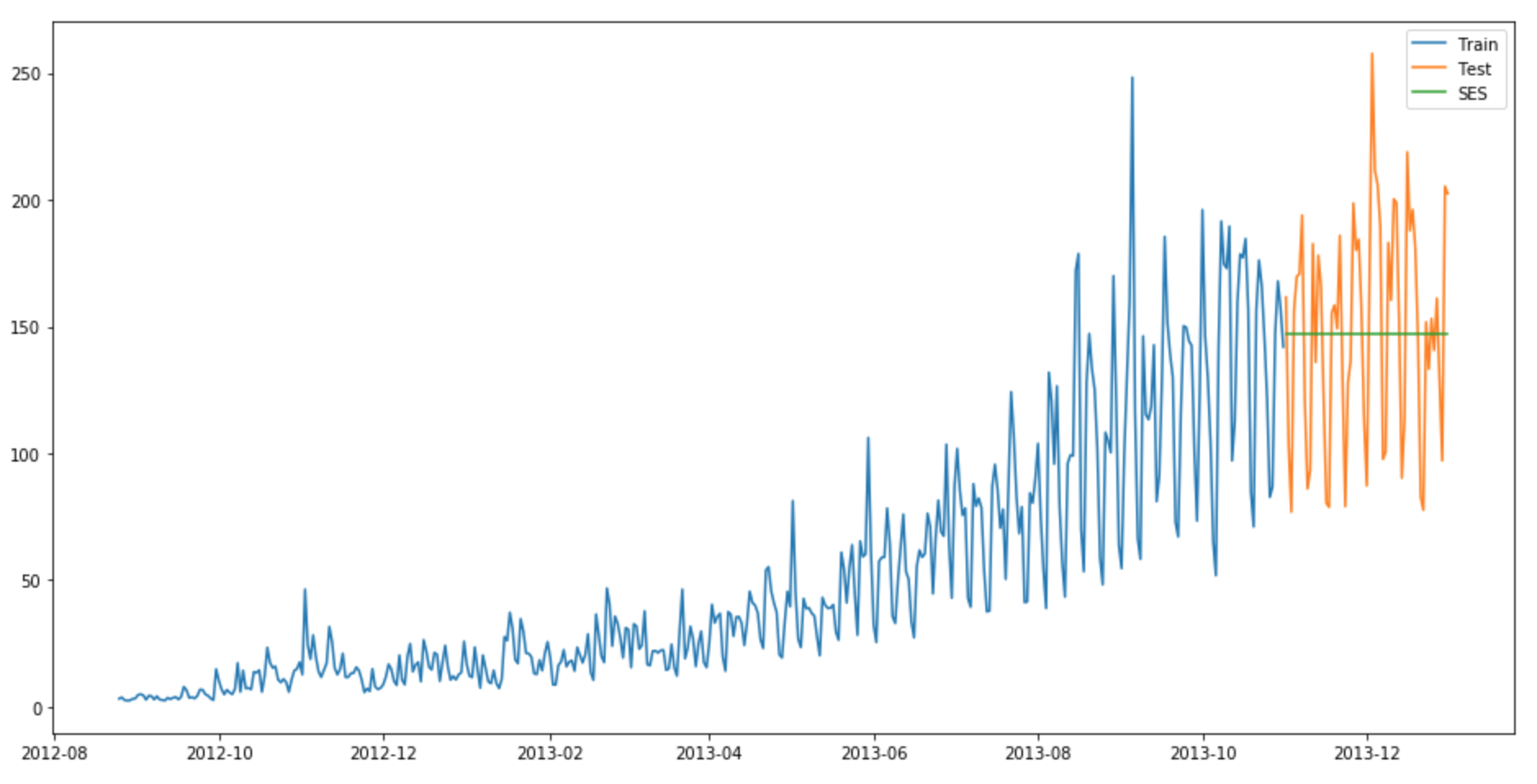We will now calculate RMSE to check to accuracy of our model.

rms = sqrt(mean_squared_error(test.Count, y_hat_avg.SES))
print(rms)

RMSE = 43.3576252252

We can see that implementing Simple exponential model with alpha as 0.6 generates a better model till now. We can tune the parameter using the validation set to generate even a better Simple exponential model.

## Method 5: Holt’s Linear Trend method

We have now learnt several methods to forecast but we can see that these models don’t work well on data with high variations. Consider that the price of the bitcoin is increasing.

If we use any of the above methods, it won’t take into account this trend. Trend is the general pattern of prices that we observe over a period of time. In this case we can see that there is an increasing trend.

Although each one of these methods can be applied to the trend as well.  E.g. the Naive method would assume that trend between last two points is going to stay the same, or we could average all slopes between all points to get an average trend, use a moving trend average or apply exponential smoothing.

But we need a method that can map the trend accurately without any assumptions. Such a method that takes into account the trend of the dataset is called Holt’s Linear Trend method.

Each Time series dataset can be decomposed into it’s componenets which are Trend, Seasonality and Residual. Any dataset that follows a trend can use Holt’s linear trend method for forecasting.

import statsmodels.api as sm
sm.tsa.seasonal_decompose(train.Count).plot()
plt.show()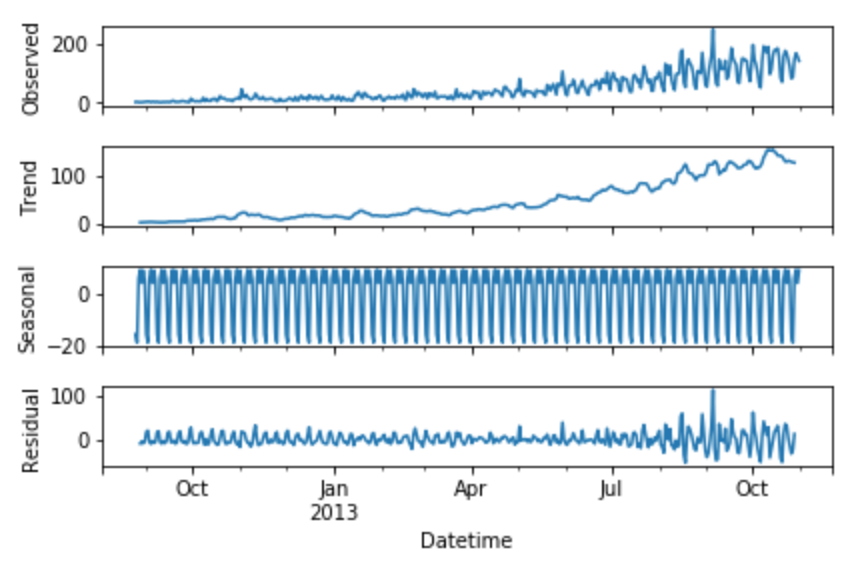We can see from the graphs obtained that this dataset follows an increasing trend. Hence we can use Holt’s linear trend to forecast the future prices.

Holt extended simple exponential smoothing to allow forecasting of data with a trend. It is nothing more than exponential smoothing applied to both level(the average value in the series) and trend. To express this in mathematical notation we now need three equations: one for level, one for the trend and one to combine the level and trend to get the expected forecast ŷ

The values we predicted in the above algorithms are called Level. In the above three equations, you can notice that we have added level and trend to generate the forecast equation.

As with simple exponential smoothing, the level equation here shows that it is a weighted average of observation and the within-sample one-step-ahead forecast The trend equation shows that it is a weighted average of the estimated trend at time t based on ℓ(t)ℓ(t1) and b(t1), the previous estimate of the trend.

We will add these equations to generate Forecast equation. We can also generate a multiplicative forecast equation by multiplying trend and level instead of adding it. When the trend increases or decreases linearly, additive equation is used whereas when the trend increases of decreases exponentially, multiplicative equation is used.Practice shows that multiplicative is a more stable predictor, the additive method however is simpler to understand.

source

y_hat_avg = test.copy()

fit1 = Holt(np.asarray(train['Count'])).fit(smoothing_level = 0.3,smoothing_slope = 0.1)
y_hat_avg['Holt_linear'] = fit1.forecast(len(test))

plt.figure(figsize=(16,8))
plt.plot(train['Count'], label='Train')
plt.plot(test['Count'], label='Test')
plt.plot(y_hat_avg['Holt_linear'], label='Holt_linear')
plt.legend(loc='best')
plt.show()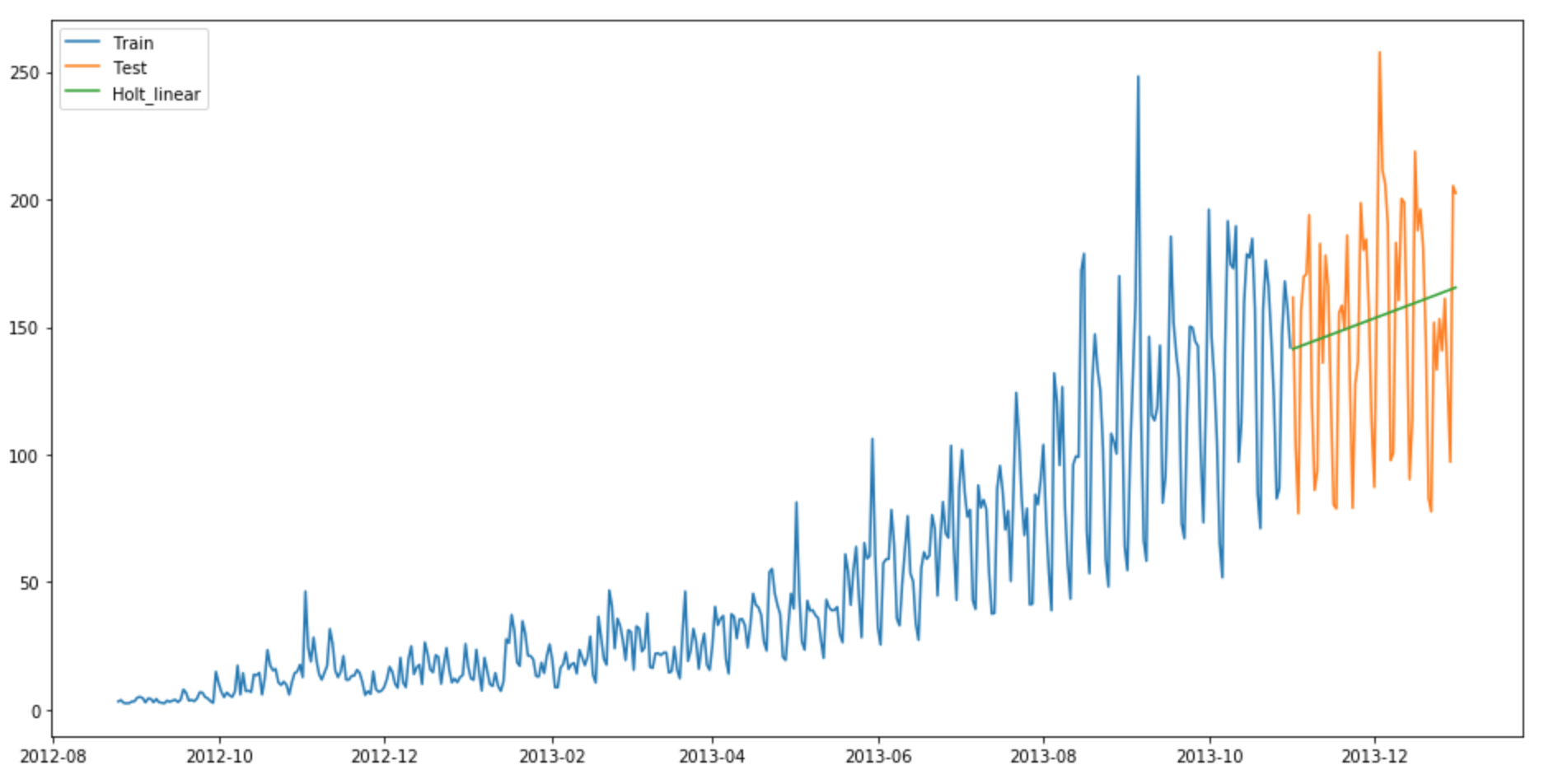We will now calculate RMSE to check to accuracy of our model.

rms = sqrt(mean_squared_error(test.Count, y_hat_avg.Holt_linear))
print(rms)
RMSE = 43.0562596115

We can see that this method maps the trend accurately and hence provides a better solution when compared with above models. We can still tune the parameters to get even a better model.

## Method 6: Holt-Winters Method

So let’s introduce a new term which will be used in this algorithm. Consider a hotel located on a hill station. It experiences high visits during the summer season whereas the visitors during the rest of the year are comparatively very less. Hence the profit earned by the owner will be far better in summer season than in any other season. This pattern will repeat itself every year. Such a repetition is called Seasonality. Datasets which show a similar set of pattern after fixed intervals of a time period suffer from seasonality.

source

The above mentioned models don’t take into account the seasonality of the dataset while forecasting. Hence we need a method that takes into account both trend and seasonality to forecast future prices. One such algorithm that we can use in such a scenario is Holt’s Winter method. The idea behind triple exponential smoothing(Holt’s Winter) is to apply exponential smoothing to the seasonal components in addition to level and trend.

Using Holt’s winter method will be the best option among the rest of the models beacuse of the seasonality factor. The Holt-Winters seasonal method comprises the forecast equation and three smoothing equations — one for the level ℓt, one for trend bt and one for the seasonal component denoted by st, with smoothing parameters α, β and γ.

source

where s is the length of the seasonal cycle, for 0 ≤ α ≤ 1, 0 ≤ β ≤ 1 and 0 ≤ γ ≤ 1.

The level equation shows a weighted average between the seasonally adjusted observation and the non-seasonal forecast for time t. The trend equation is identical to Holt’s linear method. The seasonal equation shows a weighted average between the current seasonal index, and the seasonal index of the same season last year (i.e., s time periods ago).

In this method also, we can implement both additive and multiplicative technique.  The additive method is preferred when the seasonal variations are roughly constant through the series, while the multiplicative method is preferred when the seasonal variations are changing proportional to the level of the series.

y_hat_avg = test.copy()
y_hat_avg['Holt_Winter'] = fit1.forecast(len(test))
plt.figure(figsize=(16,8))
plt.plot( train['Count'], label='Train')
plt.plot(test['Count'], label='Test')
plt.plot(y_hat_avg['Holt_Winter'], label='Holt_Winter')
plt.legend(loc='best')
plt.show()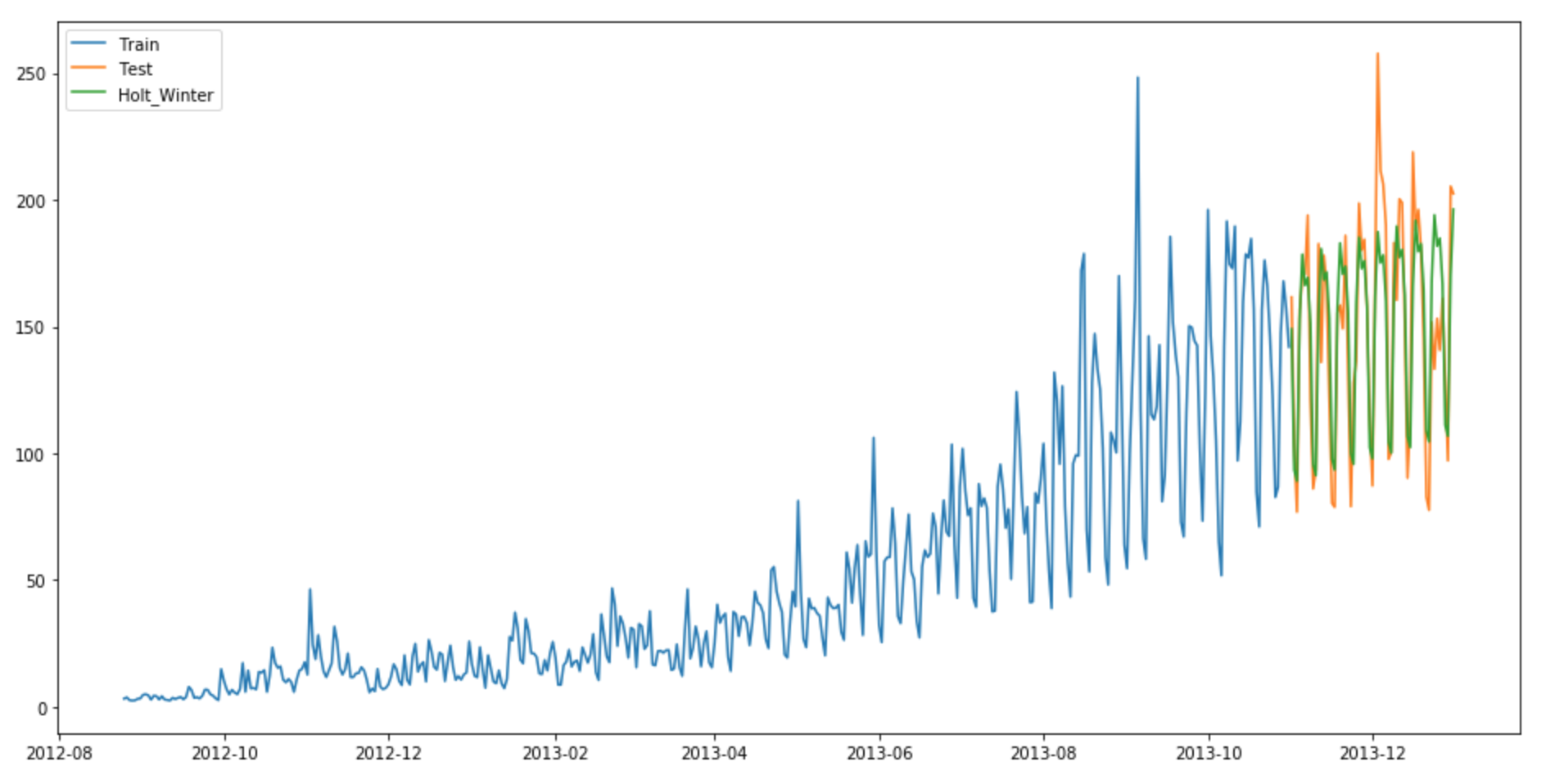We will now calculate RMSE to check to accuracy of our model.

rms = sqrt(mean_squared_error(test.Count, y_hat_avg.Holt_Winter))
print(rms)

RMSE = 23.9614925662

We can see from the graph that mapping correct trend and seasonality provides a far better solution. We chose seasonal_period = 7 as data repeats itself weekly. Other parameters can be tuned as per the dataset. I have used default parameters while building this model. You can tune the parameters to achieve a better model.

## Method 7: ARIMA

Another common Time series model that is very popular among the Data scientists is ARIMA. It stand for Autoregressive Integrated Moving average. While exponential smoothing models were based on a description of trend and seasonality in the data, ARIMA models aim to describe the correlations in the data with each other. An improvement over ARIMA is Seasonal ARIMA. It takes into account the seasonality of dataset just like Holt’ Winter method. You can study more about ARIMA and Seasonal ARIMA models and it’s pre-processing from these articles (1) and (2).

y_hat_avg = test.copy()
fit1 = sm.tsa.statespace.SARIMAX(train.Count, order=(2, 1, 4),seasonal_order=(0,1,1,7)).fit()
y_hat_avg['SARIMA'] = fit1.predict(start="2013-11-1", end="2013-12-31", dynamic=True)
plt.figure(figsize=(16,8))
plt.plot( train['Count'], label='Train')
plt.plot(test['Count'], label='Test')
plt.plot(y_hat_avg['SARIMA'], label='SARIMA')
plt.legend(loc='best')
plt.show()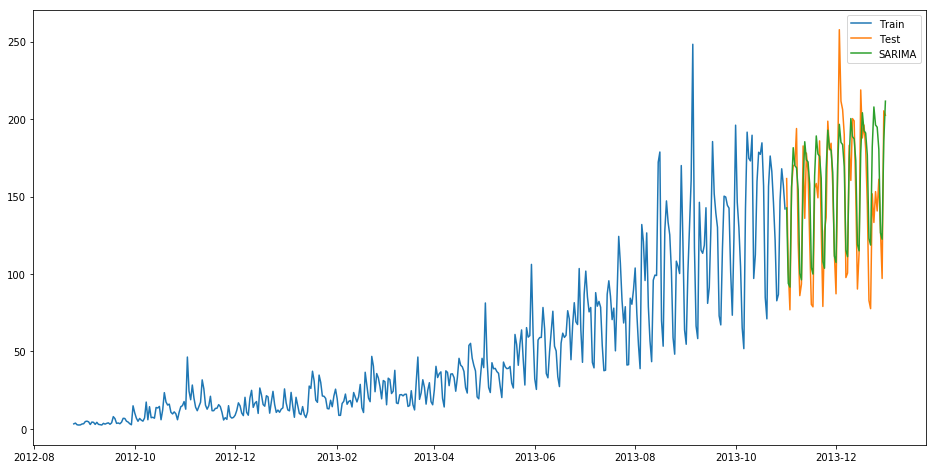We will now calculate RMSE to check to accuracy of our model.

rms = sqrt(mean_squared_error(test.Count, y_hat_avg.SARIMA))
print(rms)

RMSE = 26.035582877

We can see that using Seasonal ARIMA generates a similar solution as of Holt’s Winter. We chose the parameters as per the ACF and PACF graphs. You can learn more about them from the links provided above. If you face any difficulty finding the parameters of ARIMA model, you can use auto.arima implemented in R language. A substitute of auto.arima in Python can be viewed here.

We can compare these models on the basis of their RMSE scores.

## Projects

Now, its time to take the plunge and actually play with some other real datasets. So are you ready to take on the challenge? Test the techniques discussed in this post and accelerate your learning in Time Series Analysis with the following Practice Problem:

Q1. What is seasonal naive forecasting in python?

A. Seasonal naive forecasting in Python is a simple time series forecasting method that uses the last observed value from the same season in the previous year as the prediction for the current season. It assumes that historical patterns repeat annually. This approach can be implemented using libraries like pandas and scikit-learn, making it straightforward to apply in Python.

Q2. What is moving average time series forecasting in python?

A. Moving average time series forecasting in Python involves calculating the average of a specified number of previous observations to predict future values. This method smooths out short-term fluctuations, making it useful for identifying trends. Python libraries like pandas and NumPy provide functions to calculate moving averages, making it easy to implement this forecasting technique in Python.

## End Notes

I hope this article was helpful and now you’d be comfortable in solving similar Time series problems. I suggest you take different kinds of problem statements and take your time to solve them using the above-mentioned techniques. Try these models and find which model works best on which kind of Time series data.

One lesson to learn from these steps is that each of these models can outperform others on a particular dataset. Therefore it doesn’t mean that one model which performs best on one type of dataset will perform the same for all others too.

You can also explore forecast package built for Time series modelling in R language. You may also explore Double seasonality models from forecast package. Using double seasonality model on this dataset will generate even a better model and hence a better score.

### Learn, engage, hack and get hired!###### Gurchetan1000 Singh

Budding Data Scientist from MAIT who loves implementing data analytical and statistical machine learning models in Python. I also understand big data technology like Hadoop and Alteryx.

## 75 thoughts on "7 methods to perform Time Series forecasting (with Python codes)"###### Rodrigo Esquivel says:February 08, 2018 at 10:36 am
Hello Gurchetan, Thks for your interesting article. Even though I use R, I think the question is interesting for any user of Time series regarding of the tool used. I implemented for a client a Time Series using Holt -Winters. Everything was fine, but because my client is not an IT or stats proficient guy I needed to provide among the implementation some kind of algorythm that could calculate for him the 3 coeffcients used in the Holt Winters method. My first solution was very simple just generate a random set of numbers and the one that has the least SSE is the one the system would use to forecast the Timeseries. At this moment is working fine but I would like to optimize it using for example Nelder-Mead. Because the environment is not R pure, not all the libraries are recognized meaning that I would have to code the whole Nelder-Mead function. My question is acording to your experience is worth the effort? Would my algorythm would gain performance and/or accuracy? Thks a lot for your time. Reply###### Fawad Mahdi says:February 08, 2018 at 11:01 am###### Kotrappa Sirbi says:February 08, 2018 at 12:24 pm
Nice very useful article for time series beginners, Thanks Reply###### Alain Craven says:February 08, 2018 at 12:35 pm
I am getting a range of errors when running the code. Some are easier to fix, but this one is stopping me: ValueError: Start must be in dates. Got 2013-11-1 | 2013-11-01 00:00:00 Reply###### Gurchetan Singh says:February 08, 2018 at 1:39 pm
Hey Alain Which part of the code produced this error ? Reply###### Ritesh Garg says:February 08, 2018 at 3:40 pm
Hi Admin, There is an errors in the at the subsetting & aggregating part. PFB the corrected code:#Aggregating the dataset at daily level df.Timestamp = pd.to_datetime(df.Datetime,format='%d-%m-%Y %H:%M') df.index = df.Timestamp df = df.resample('D').mean() #Creating train and test set #Index 10392 marks the end of October 2013 train=df[0:433] test=df[433:] Reply###### Ankit says:February 08, 2018 at 7:19 pm
Gurchetan , thanks for your wonderful article. Can we use time series prediction with set of data say train timings, we have N number of trains. their past history of arrival is there with us. some days it is running late, on time etc. So we perdict train XYZ will reach station swd at this time tomorrow? i am looking for similar kind of time series prediction code. Can you help me by providing some insight. Thank you Reply###### Gianni says:February 08, 2018 at 7:27 pm
Great article ! Just i want to punctualize that on kaggle/python docker container, Jupyter, doesn't work because exponentialsmoothing is too much recent. I tried to install from the master using this instruction: !pip install git+https://github.com/statsmodels/statsmodels.git Kudos to: https://stackoverflow.com/questions/48646481/python-statsmodels-and-simple-exponential-smoothing-in-jupyter-and-pycharm But anyway it doesn't work, wondering why ? Reply###### Wagner Rodeski says:February 08, 2018 at 10:15 pm###### Bruno Nazário says:February 09, 2018 at 1:51 am
Hello! Great article and explanation! I've tried the above mentioned method, but coudn't find ExponentialSmoothing, SimpleExpSmoothing, Holt classes under the statsmodels.tsa.api package. Any tips? Reply###### ch swamy says:February 09, 2018 at 11:38 am
Hi Team, the aggregation section , you have mentioned the following #Aggregating the dataset at daily level df.Timestamp = pd.to_datetime(df.Datetime,format='%d-%m-%Y %H:%M') df.index = df.Timestamp df = df.resample('D').mean() If you are aggregating the data from Hours to Days.. then why do you consider / take the mean of resample . I think it should be resample of "Addition" .... i.e df= df.resample('D').sum() Hourly commuters are added up for a Day .. so on the Day you will have a cumilative number of commuters. please correct me if i am wrong .. Reply###### Oxana Gafaiti says:February 09, 2018 at 12:01 pm###### Gurchetan Singh says:February 09, 2018 at 3:39 pm###### Marcelle says:February 09, 2018 at 4:09 pm
I had the same error and putting it in double quotes sorted it, e.g. start="2013-01-01". Maybe try that. Reply###### Gurchetan Singh says:February 09, 2018 at 6:21 pm
Hey Bruno I have added an extra section indicating the steps to install statsmodel correctly. I hope it helps. Reply###### Gurchetan Singh says:February 09, 2018 at 6:23 pm###### Gurchetan Singh says:February 09, 2018 at 6:26 pm
Hey Ritesh I updated the ordering of the sections at the last moment which led to this. Updated the order. Thanks for correcting. Reply###### Gurchetan Singh says:February 09, 2018 at 6:28 pm###### Gurchetan Singh says:February 09, 2018 at 7:16 pm
Hey Oxana You can visit the link and register for the Practice problem. After registering, you can view the dataset under Data tab. Reply###### Mario says:February 10, 2018 at 12:00 am
Nice article, thanks for sharing! Please note that you might need some dependencies for statsmodels to build it from source. In my case cython was missing. More details can be found here: http://www.statsmodels.org/dev/install.html Reply###### Gurchetan Singh says:February 12, 2018 at 12:12 pm
Hey Resample is used to aggregate the hourly counts of a Day. If we use resample().sum(), it will add all the hourly Counts and if we use resample().mean() it will calculate the mean hourly Count of that day. Hence we can use any one of these methods to resample. Reply###### Gurchetan Singh says:February 12, 2018 at 12:19 pm
Hey Mario Thank you for this information. Reply###### Gurchetan Singh says:February 12, 2018 at 12:43 pm
Hey Ankit We can solve such problems with Time series models with but such data would involve multiple seasons. Consider winter season for example, there is a high chance that that train would arrive late due to fog, smog, etc. Same scenes during festival time periods. So I dont think there would be much issue in solving such problems. Do contact me if you face any difficulty in solving and implementing these methods. Cheers Reply###### Gurchetan Singh says:February 12, 2018 at 12:53 pm
Hey Rodrigo I have implemented these algorithms many times just on practice datasets and never for any company/client and using SSE minimisation always works for me. Though I havent tried Nelder-mead function, but you can always try and implement it. I assume it will only increase your accuracy and performance but if it doesn't, you will anyhow learn something new developing it. So do make a try and check if it is worthy or not. Do update me with the results. Cheers Reply###### Ritesh Garg says:February 13, 2018 at 9:59 pm
Thanks for updating the code. BTW this is really helpful :) Reply###### Dominic Yang says:February 15, 2018 at 12:39 am
Hey, it's a great article! I had an error about plotting data below. AttributeError: 'numpy.float64' object has no attribute 'plot' --------------------- #Plotting data --> train.Count.plot(figsize=(15,8), title= 'Daily Ridership', fontsize=14) test.Count.plot(figsize=(15,8), title= 'Daily Ridership', fontsize=14) plt.show() -------------------- I've searched and tried but couldn't solve it. Do you have any advice? Thanks. Reply###### Gurchetan Singh says:February 15, 2018 at 12:47 pm
Hey Try following the solution given on https://stackoverflow.com/questions/27744963/numpy-float64-object-has-no-attribute-plot Reply###### Umesh Baraskar says:February 18, 2018 at 6:07 pm
Hello Sir, Could I get data which you have used to analysis purpose ? Number passengers that data which you have introduced while introducing the Time series. Reply###### Vivek says:February 27, 2018 at 3:34 pm
From where I can get dataset? Reply###### Amogha says:February 27, 2018 at 10:56 pm
Hello Gurchetan Singh! I'm an engineering student practicing ML & DL from past one year & the methods you have illustrated here are very nice and useful. I'll be very happy to connect to you via LinkedIn. Please share your profile Thanks a lot for this article!! Reply###### Gurchetan Singh says:February 27, 2018 at 11:05 pm###### Gurchetan Singh says:February 27, 2018 at 11:11 pm###### Subodh says:March 02, 2018 at 3:24 pm
Hi Gurcharan I dont see Holt as part of the statsmodels , please clarify Reply###### Subodh says:March 02, 2018 at 3:26 pm
Below code produces error from statsmodels.tsa.api import ExponentialSmoothing, SimpleExpSmoothing, Holt Reply###### maria says:March 09, 2018 at 9:50 pm
Thanks for the information. It was very helpful. Just one question. Is it possible to build prediction intervals for holt winters in python? Reply###### Karthik says:March 19, 2018 at 10:08 pm
Wonderful article on the forecasting techniques. One small point of feedback though - can you please fix the grammatical errors the article is replete with, otherwise, it would make for a perfect article. Minor grammatical nits have made me go over this article several times to make good sense. Hope the feedback will be taken all in good spirit :) Reply###### Faizan Shaikh says:March 20, 2018 at 1:15 pm
Thanks for the feedback Karthik Reply###### Gurchetan Singh says:March 20, 2018 at 2:39 pm###### Gurchetan Singh says:March 20, 2018 at 2:41 pm###### GD says:April 04, 2018 at 7:49 pm
Hi Gurchetan, Really nice article. Thanks a lot for taking the time to document this. It helped me. I have a question regarding Holt Winter's. Can you please help? For Holt Winter's you used: fit1 = ExponentialSmoothing(np.asarray(train['Count']) ,seasonal_periods=7 ,trend='add', seasonal='add',).fit() Is there a way where we can change the alpha, beta and gamma values? Does the 'add' option optimise these values using a grid search? If yes, then can we access the optimised values? Thank you. Reply###### will says:April 05, 2018 at 4:48 pm
Going through this article for a work project and although I haven't finished testing all the code yet, I found a method to make a vectorized moving average that actually moves alongside the trend. This StackOverflow answer shows how to calculate MA with Pandas: https://stackoverflow.com/questions/14313510/how-to-calculate-moving-average-using-numpy so by using this instead of the proposed MA solution, you get a graph that is more close to the actual fluctuations in the data, depending of course on the window that you choose. Hope it helps. Reply###### Gurchetan Singh says:April 06, 2018 at 1:51 pm
Hey You can definitely changes the values of the hyperparamteres. There are three hyperparameters smoothing_level, smoothing_slope and smoothing_seasonal which you can alter while fitting eg. fit(smoothing_level= _, smoothing_slope= _, smoothing_seasonal= _). I have used 'add' for trend to show the trend increases linearly as mentioned in the article. You can also use 'mul' if the trend increases exponentially. Same is the case with seasonality. Reply###### Gurchetan Singh says:April 06, 2018 at 2:02 pm
Hey Thank you for sharing. Reply###### ABIN JOHN THOMAS says:April 20, 2018 at 7:28 am
Hi, I got a warning about creating a new column to the dataframe by using dot operator. This is the line of code: df.Timestamp = pd.to_datetime(df.Datetime,format='%d-%m-%Y %H:%M') But this created the column. When I replaced the dot operator with square brackets, there was no warning. here is my code: df["Timestamp"] = pd.to_datetime(df.Datetime,format='%d-%m-%Y %H:%M') I am using windows 7, running notebook on Pycharm with python 3.6. Can you please let me know why system is throwing the warning, Is it because I used a different python version? Or is it a recent change to pandas where they don't allow to create new column through dot operator. Thanks Abin Reply###### Mateusz Polnik says:May 11, 2018 at 1:59 pm
Hi Gruchetan, The formula for weighted moving average should not have the 1/m factor, because we assume that all weights add up to 1. Overall, Great article! It is a coherent story that is enjoyable to read. Reply###### Souvik Ray says:May 14, 2018 at 4:44 pm
Hey, I have a small doubt.In the average and moving average technqiue, The 'train' and 'test' that you mention, is it the original train and test that has been sliced out of df or is it train = train.resample('D').mean() and test = test.resample('D').mean() respectively? Reply###### Pulkit Sharma says:May 15, 2018 at 6:44 pm
Hi Souvik, The train and test are the original train and test that has been sliced out of df. Reply###### Pulkit Sharma says:May 15, 2018 at 6:49 pm
Hi ABIN, Instead of using "Timestamp" try to use 'Timestamp'. Using df['Timestamp'] = pd.to_datetime(df.Datetime,format=’%d-%m-%Y %H:%M’) would give you the result. Reply###### Alex says:May 29, 2018 at 8:37 pm
Could you take a look here: https://stats.stackexchange.com/questions/348803/strange-output-while-using-holt-s-linear-trend-method ? Reply###### song says:May 30, 2018 at 11:39 am###### Aishwarya Singh says:May 30, 2018 at 4:47 pm###### Aishwarya Singh says:May 30, 2018 at 6:19 pm
Hi, Did you use the same dataset as in the article? Reply###### Pedram Jahangiri says:June 01, 2018 at 11:46 pm
Hi Gurchetan: Thanks for your interesting article. I am also interested in time series forecasting with features. Basically building models based on X features and prediction Y, Y=f(X). Let's say you have time series of electric consumption and you want to predict that based on actual weather data and day type. Can you make comment on this. Thansk Reply###### Karthik Rampelli says:June 06, 2018 at 2:32 pm###### rachit says:June 12, 2018 at 6:36 pm
Hey how can we use this to predict future values? Great article though!! Reply###### Aishwarya Singh says:June 18, 2018 at 10:25 pm###### Sriram says:June 19, 2018 at 12:16 pm###### Aishwarya Singh says:June 20, 2018 at 8:36 am
Hi, If you are able to fit your training using any of the model, you can simply use .predict to make the predictions. Have a look at this course, it will help you understand how to predict future values: Time Series forecasting using python Reply###### Aishwarya Singh says:June 20, 2018 at 8:45 am###### Aishwarya Singh says:June 20, 2018 at 8:53 am
Hi, This is an interesting problem. I would suggest you to share the problem on the discuss portal so that the community can help you. Here is the link : https://discuss.analyticsvidhya.com/ Reply###### Rodrigo Esquivel says:June 21, 2018 at 11:48 am###### Ayush Rastogi says:June 25, 2018 at 12:57 am
URL for practice problem : https://datahack.analyticsvidhya.com/contest/practice-problem-time-series-2/ Reply###### Marina says:June 29, 2018 at 5:42 am
Great article! I can't get it to work for some reason. Both, the Holt, and SARIMA, come out flattened to a tiny range (like between 46.0 and 46.5) whereas the original data goes from 10 to 50. Could you please tell me what might be going wrong? Reply###### Aishwarya Singh says:June 29, 2018 at 11:18 am
Hi Marina, This might be because of the parameters you have chosen. Try setting different values for p,q. Reply###### Kairos says:July 09, 2018 at 3:11 pm
Great article- Thanks for your time and effort. I got a problem, and is the following: I can't find the link to download the dataset. I've alreade logged in but or I'm crazy or the link doesn't appear anywhere. Can you type it for me, please? Reply###### Aishwarya Singh says:July 10, 2018 at 12:08 pm###### Harshal says:August 07, 2018 at 4:44 pm
Hello guys.Can any one please let me know how to deal with daily data.If some one can share me the code for daily data would be really helpful. Reply###### Pulkit Sharma says:August 07, 2018 at 8:20 pm
Hi Harshal, You can refer this course on time series analysis. In this course the hourly data has been converted to daily data and models have been built on the same. Reply###### Andyd says:August 17, 2018 at 11:22 am
Where is the link to the train dataset, I cant get assess to it Reply###### Pulkit Sharma says:August 17, 2018 at 11:52 am###### Divya says:August 21, 2018 at 4:27 am
Hi, What if my dataset depended on multiple input parameters. How do I go about applying ARIMA model on such a dataset? Looking forward to your reply. Thanks. Reply###### Aishwarya Singh says:August 21, 2018 at 10:13 am
Hi Divya, For datasets with have multiple input values (apart from the time variable) you will have to use algorithms like linear regression or random forest. Reply###### Rajnish Kumar says:September 05, 2018 at 3:33 pm
getting error at df.Timestamp = pd.to_datetime(df.Datetime,format='%Y') error is AttributeError: 'DataFrame' object has no attribute 'Datetime' my data looks like below 211 2018 25.600000 212 2018 36.501000 213 2018 32.875000 214 2018 30.900000 215 2018 32.000000 216 2018 35.000000 and my code is train=df[0:187] test=df[187:] df.Timestamp = pd.to_datetime(df.Datetime,format='%Y') df.index = df.Timestamp df = df.resample('D').mean() train.Timestamp = pd.to_datetime(train.Datetime,format='%Y') train.index = train.Timestamp train = train.resample('D').mean() test.Timestamp = pd.to_datetime(test.Datetime,format='%Y') test.index = test.Timestamp test = test.resample('D').mean() plt.plot(train) plt.plot(test,color='red') plt.show() y_hat_avg = test.copy() fit1 = ExponentialSmoothing(np.asarray(train['Rate']) ,seasonal_periods=7 ,trend='add', seasonal='add',).fit() y_hat_avg['Holt_Winter'] = fit1.forecast(len(test)) plt.figure(figsize=(16,8)) plt.plot( train['Rate'], label='Train') plt.plot(test['Rate'], label='Test') plt.plot(y_hat_avg['Holt_Winter'], label='Holt_Winter') plt.legend(loc='best') plt.show() rms = sqrt(mean_squared_error(test.Count, y_hat_avg.Holt_Winter)) print(rms) please help Reply###### Aishwarya Singh says:September 05, 2018 at 7:33 pm
Hi, you the following code  df.Timestamp = pd.to_datetime(df[columnname],format='%Y') . Here df will be your dataframe and columnname should be the name of column which has the date values. Reply###### Nayak says:September 11, 2018 at 4:36 pm
Very nice article with a great explanation. Eventhough ARIMA is smilar to Holt-Winters Method. But further explanation on ARIMA would have helped. Reply